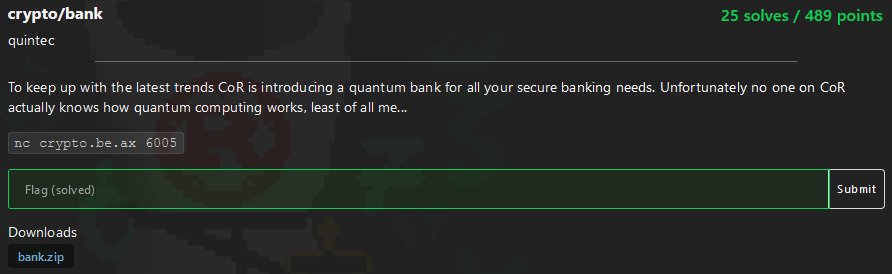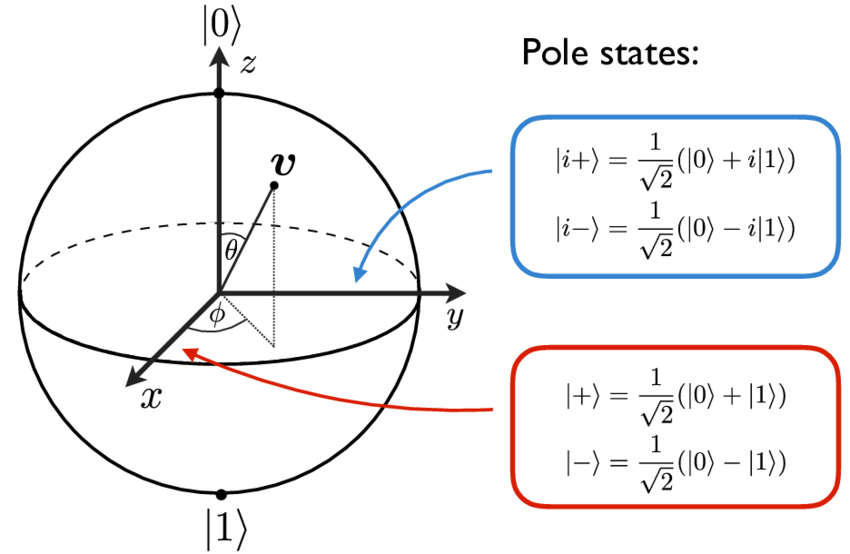# corCTF 2021 - bank

Cryptography – 489 pts (25 solves) – Chall author: quintec

A new and upcoming bank called ‘CoR Bank’ is using the most recent cryptographic security, quantum cryptography! We even get a whole free dollar :). There is only one small problem for them, we are allowed to fidget with our qubits. And as usual, we are up to no good.

Check out write-ups by my teammates on K3RN3L4RMY.com

## ExplorationUpon registering a new account with the bank, they assign a ‘bill’ to us that consists of 50 qubits. All of them have been set up in one of four states: ‘0’ $[1,0]$, ‘1’ $[0,1]$, ‘+’ $[1,1]/\sqrt{2}$, and ‘-‘ $[1,-1]/\sqrt{2}$. The bank will let us buy the flag if we can figure out the configuration of our ‘bill’.

Brute-forcing is no good here as the possible key space is 4**50, so what can we do? Well luckily for us, the bank allows us to fidget with each of our qubits. More specifically, we can apply the following transformations:

1. Apply X gate
2. Apply Y gate
3. Apply Z gate
5. Apply rotation gate
6. Measure qubit in ‘01’ basis
7. Measure qubit in ‘+-‘ basis
8. Verify qubit

Options 1-4 are trivial and not too interesting. Options 6 and 7 allow us to measure and collapse the state of the qubit in one of the two basis. In other words, the measurement is done on our actual qubit and not on a fake/copy. Option 8 will ‘verify’ our qubit: the server will measure, and collapse, the state of our qubit in the base it was made in. If it measures the same state as it was originally put in (the state in the bill) it succesfully verifies, otherwise it fails. But the most freedom is given to us by option 5, which allows us to rotate our qubit with any angle we want.

Because measuring the qubit will collapse it, we should only measure it once we know for sure in what basis to measure. If we just guess the original base at 50% success rate, the probability of guessing our bill correctly is 0.5**50. Still way too unlikely. So before we do any measurements, we have to figure out its base. But how?

## Exploitation

Because of the amount of freedom we are given with our qubits, it would not surprise me if there are multiple correct solutions to retrieve the flag. So make sure to check out other write-ups too! However, my eyes immediately fell on the roation gate. Taking a look at the source code we see it is actually a Rz gate, a rotation gate around the z-axis. Now this is interesting, as the z-axis is parallel to the ‘01’ base and perpendicular to the ‘+-‘ base, see this Bloch sphere (source: A. Ketterer, 2016).If we now apply a half-rotation, so $\pi$ radians, we find only a state-flip in the ‘+-‘ base!

$\mathrm{Rot_z}(\left|0\right\rangle, \pi) = \begin{bmatrix} 1 & 0 \\ 0 & -1 \end{bmatrix} \begin{bmatrix} 1 \\ 0 \end{bmatrix} = \begin{bmatrix} 1 \\ 0 \end{bmatrix} = \left|0\right\rangle$ $\mathrm{Rot_z}(\left|1\right\rangle, \pi) = \begin{bmatrix} 1 & 0 \\ 0 & -1 \end{bmatrix} \begin{bmatrix} 0 \\ 1 \end{bmatrix} = \begin{bmatrix} 0 \\ -1 \end{bmatrix} = \left|1\right\rangle$ $\mathrm{Rot_z}(\left|+\right\rangle, \pi) = \begin{bmatrix} 1 & 0 \\ 0 & -1 \end{bmatrix} \frac{1}{\sqrt{2}} \begin{bmatrix} 1 \\ 1 \end{bmatrix} = \frac{1}{\sqrt{2}}\begin{bmatrix} 1 \\ -1 \end{bmatrix} = \left|-\right\rangle$ $\mathrm{Rot_z}(\left|-\right\rangle, \pi) = \begin{bmatrix} 1 & 0 \\ 0 & -1 \end{bmatrix} \frac{1}{\sqrt{2}} \begin{bmatrix} 1 \\ -1 \end{bmatrix} = \frac{1}{\sqrt{2}}\begin{bmatrix} 1 \\ 1 \end{bmatrix} = \left|+\right\rangle$

So if we rotate our qubit by $\pi$ radians and follow-up with a verify, the server will measure our qubit in its original base. Since the rotation only resolves in flip in the ‘+-‘ base such that its verification will fail, it keeps the ‘01’ base intact such that its verification will succeed. Hence depending on the succes of the verification we work out the original base of the qubit.

Knowing the correct base for our qubit, we simply measure it ourselves in said base. We should keep in mind we flipped the ‘+-‘ base though, so if we measure in this base, we should flip its outcome to find the corresponding state as in our bill. Alright, let’s pwn this server!

#!/usr/bin/env python3
#
# Polymero
#

# Imports
from pwn import *
from numpy import pi

# Remote connection
host = "crypto.be.ax"
port = 6005
s = remote(host,port)
context.log_level = 'debug'

# Get past entry screen
s.recv()
s.sendline(b'y')
s.recv()

# Recover bill by fidgeting with our qubits
bill = ''

for k in range(50):

s.sendline(b'1')
s.recv()

# Select qubit
s.sendline(str(k).encode())
s.recv()

s.sendline(b'5')
s.recv()
s.sendline(str(pi).encode())
s.recv()

# Verify qubit
s.sendline(b'8')
if s.recvuntil(b'\n',drop=True) == b'Qubit successfully verified.':

# Measure in '01' base
s.recv()
s.sendline(b'6')

# Add qubit state to our bill
bill += s.recvuntil(b'\n',drop=True).decode()[-1]

else:

# Measure in '+-' base
s.recv()
s.sendline(b'7')

# Add flipped qubit state to our bill
flip = s.recvuntil(b'\n',drop=True).decode()[-1]
pmdic = { '+' : '-', '-' : '+' }
bill += pmdic[flip]

s.recv()

# Go back
s.sendline(b'9')

s.recv()

# Turn in bill
s.sendline(b'2')
s.recv()
s.sendline(bill.encode())

# Get flag
s.recv()
s.recv()
s.recv()


Ta-da!

corctf{4lw4ys_d3str0y_y0ur_f4k3s}